Home > PC > Chapter 11 > Lesson 11.2.1 > Problem11-75

11-75.
1. Simplify the following expressions into a single trigonometric function. Homework Help ✎

1. cosacosb − sinasinb

2. 20 sin(3x) cos(3x)

3. sin(3x) cos(x) − cos(3x) sin(x)

4. sin2(4y) − cos2(4y)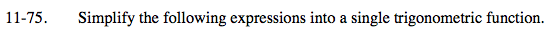Use the Angle Sum and Difference Identities.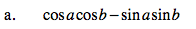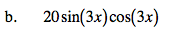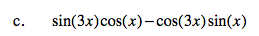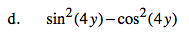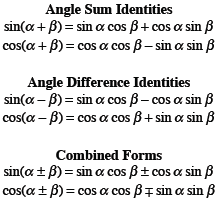This expression is equivalent to −[cos(4y)cos(4y) − sin(4y)sin(4y)].